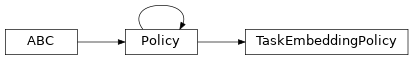# garage.tf.policies.task_embedding_policy¶

Policy class for Task Embedding envs.

class TaskEmbeddingPolicyBase class for Task Embedding policies in TensorFlow.

This policy needs a task id in addition to observation to sample an action.

property encoder(self)

garage.tf.embeddings.encoder.Encoder: Encoder.

get_latent(self, task_id)

Get embedded task id in latent space.

Parameters

task_id (np.ndarray) – One-hot task id, with shape $$(N, )$$. N is the number of tasks.

Returns

An embedding sampled from embedding distribution, with

shape $$(Z, )$$. Z is the dimension of the latent embedding.

dict: Embedding distribution information.

Return type

np.ndarray

property latent_space(self)

akro.Box: Space of latent.

property task_space(self)

akro.Box: One-hot space of task id.

property augmented_observation_space(self)

akro.Box: Concatenated observation space and one-hot task id.

property encoder_distribution(self)

tfp.Distribution.MultivariateNormalDiag: Encoder distribution.

abstract get_action(self, observation)

Get action sampled from the policy.

Parameters

observation (np.ndarray) – Augmented observation from the environment, with shape $$(O+N, )$$. O is the dimension of observation, N is the number of tasks.

Returns

Action sampled from the policy,

with shape $$(A, )$$. A is the dimension of action.

dict: Action distribution information.

Return type

np.ndarray

abstract get_actions(self, observations)

Get actions sampled from the policy.

Parameters

observations (np.ndarray) – Augmented observation from the environment, with shape $$(T, O+N)$$. T is the number of environment steps, O is the dimension of observation, N is the number of tasks.

Returns

Actions sampled from the policy,

with shape $$(T, A)$$. T is the number of environment steps, A is the dimension of action.

dict: Action distribution information.

Return type

np.ndarray

abstract get_action_given_task(self, observation, task_id)

Sample an action given observation and task id.

Parameters
• observation (np.ndarray) – Observation from the environment, with shape $$(O, )$$. O is the dimension of the observation.

• task_id (np.ndarray) – One-hot task id, with shape :math:(N, ). N is the number of tasks.

Returns

Action sampled from the policy, with shape

$$(A, )$$. A is the dimension of action.

dict: Action distribution information.

Return type

np.ndarray

abstract get_actions_given_tasks(self, observations, task_ids)

Sample a batch of actions given observations and task ids.

Parameters
• observations (np.ndarray) – Observations from the environment, with shape $$(T, O)$$. T is the number of environment steps, O is the dimension of observation.

• task_ids (np.ndarry) – One-hot task ids, with shape $$(T, N)$$. T is the number of environment steps, N is the number of tasks.

Returns

Actions sampled from the policy,

with shape $$(T, A)$$. T is the number of environment steps, A is the dimension of action.

dict: Action distribution information.

Return type

np.ndarray

abstract get_action_given_latent(self, observation, latent)

Sample an action given observation and latent.

Parameters
• observation (np.ndarray) – Observation from the environment, with shape $$(O, )$$. O is the dimension of observation.

• latent (np.ndarray) – Latent, with shape $$(Z, )$$. Z is the dimension of latent embedding.

Returns

Action sampled from the policy,

with shape $$(A, )$$. A is the dimension of action.

dict: Action distribution information.

Return type

np.ndarray

abstract get_actions_given_latents(self, observations, latents)

Sample a batch of actions given observations and latents.

Parameters
• observations (np.ndarray) – Observations from the environment, with shape $$(T, O)$$. T is the number of environment steps, O is the dimension of observation.

• latents (np.ndarray) – Latents, with shape $$(T, Z)$$. T is the number of environment steps, Z is the dimension of latent embedding.

Returns

Actions sampled from the policy,

with shape $$(T, A)$$. T is the number of environment steps, A is the dimension of action.

dict: Action distribution information.

Return type

np.ndarray

split_augmented_observation(self, collated)

Splits up observation into one-hot task and environment observation.

Parameters

collated (np.ndarray) – Environment observation concatenated with task one-hot, with shape $$(O+N, )$$. O is the dimension of observation, N is the number of tasks.

Returns

Vanilla environment observation,

with shape $$(O, )$$. O is the dimension of observation.

np.ndarray: Task one-hot, with shape $$(N, )$$. N is the number

Return type

np.ndarray

property state_info_specs(self)

State info specification.

Returns

keys and shapes for the information related to the

module’s state when taking an action.

Return type

List[str]

property state_info_keys(self)

State info keys.

Returns

keys for the information related to the module’s state

when taking an input.

Return type

List[str]

reset(self, do_resets=None)

Reset the policy.

This is effective only to recurrent policies.

do_resets is an array of boolean indicating which internal states to be reset. The length of do_resets should be equal to the length of inputs, i.e. batch size.

Parameters

do_resets (numpy.ndarray) – Bool array indicating which states to be reset.

property name(self)

Name of policy.

Returns

Name of policy

Return type

str

property env_spec(self)

Policy environment specification.

Returns

Environment specification.

Return type

garage.EnvSpec

property observation_space(self)

Observation space.

Returns

The observation space of the environment.

Return type

akro.Space

property action_space`(self)

Action space.

Returns

The action space of the environment.

Return type

akro.Space